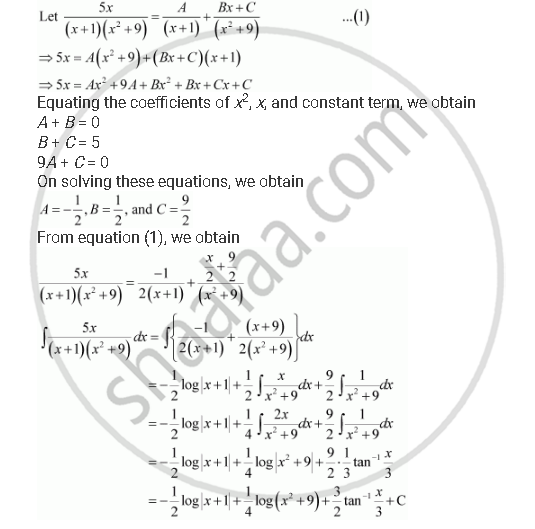Share

# Integrate the Functions (5x)/((X+1)(X^2 +9)) - CBSE (Science) Class 12 - Mathematics

ConceptIntegration as an Inverse Process of Differentiation

#### Question

Integrate the functions  (5x)/((x+1)(x^2 +9))

#### SolutionIs there an error in this question or solution?

#### Video TutorialsVIEW ALL 

Solution Integrate the Functions (5x)/((X+1)(X^2 +9)) Concept: Integration as an Inverse Process of Differentiation.
S